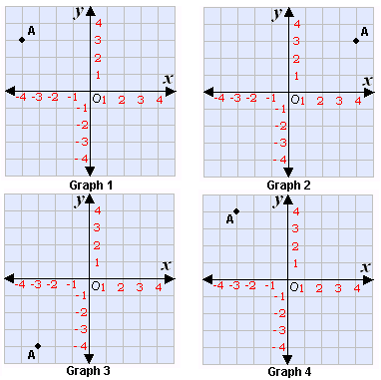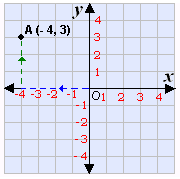## Definition Of Ordered Pair

A pair of numbers used to locate a point on a coordinate plane is called an ordered pair.

An ordered pair is written in the form (x, y) where x is the x-coordinate and y is the y-coordinate.

In three-dimensional cordinates, (x, y, z) gives the location of a point. It is called an ordered triple.
The ordered pair (5, -2) means the point is located 5 units right and 2 units down the origin.
The point of intersection of the x- and y- axes is called the origin. The ordered pair that describes the origin is (0, 0).

### Examples of Ordered Pair

Following are some examples of ordered pairs.
(5, -2), (0, 0), (2, 5), (0.3, - 0.7)

### Solved Example on Ordered Pair

#### Ques: Graph the ordered pair (- 4, 3) on the coordinate plane and call it A.##### Choices:

A. Graph 1
B. Graph 2
C. Graph 3
D. Graph 4